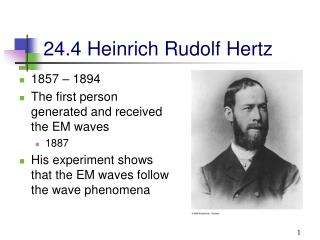DownloadDownload Presentation24.4 Heinrich Rudolf Hertz

# 24.4 Heinrich Rudolf Hertz

Download Presentation## 24.4 Heinrich Rudolf Hertz

- - - - - - - - - - - - - - - - - - - - - - - - - - - E N D - - - - - - - - - - - - - - - - - - - - - - - - - - -
##### Presentation Transcript

1. 24.4 Heinrich Rudolf Hertz • 1857 – 1894 • The first person generated and received the EM waves • 1887 • His experiment shows that the EM waves follow the wave phenomena

2. Hertz’s Experiment • An induction coil is connected to a transmitter • The transmitter consists of two spherical electrodes separated by a narrow gap to form a capacitor • The oscillations of the charges on the transmitter produce the EM waves. • A second circuit with a receiver, which also consists of two electrodes, is a single loop in several meters away from the transmitter.

3. Hertz’s Experiment, cont • The coil provides short voltage surges to the electrodes • As the air in the gap is ionized, it becomes a better conductor • The discharge between the electrodes exhibits an oscillatory behavior at a very high frequency • From a circuit viewpoint, this is equivalent to an LC circuit

4. Hertz’s Experiment, final • Hertz found that when the frequency of the receiver was adjusted to match that of the transmitter, the energy was being sent from the transmitter to the receiver • Hertz’s experiment is analogous to the resonance phenomenon between a tuning fork and another one. • Hertz also showed that the radiation generated by this equipment exhibited wave properties • Interference, diffraction, reflection, refraction and polarization • He also measured the speed of the radiation

5. LC circuit

6. 24.5 Energy Density in EM Waves • The energy density, u, is the energy per unit volume • For the electric field, uE= ½ eoE2 • For the magnetic field, uB = B2 / 2mo • Since B = E/c and

7. Energy Density, cont • The instantaneous energy density associated with the magnetic field of an EM wave equals the instantaneous energy density associated with the electric field • In a given volume, the energy is shared equally by the two fields

8. Energy Density, final • The total instantaneous energy density in an EM wave is the sum of the energy densities associated with each field • u =uE + uB = eoE2 = B2 / mo • When this is averaged over one or more cycles, the total average becomes • uav = eo (Eavg)2 = ½ eoE2max = B2max / 2mo

9. Energy carried by EM Waves • Electromagnetic waves carry energy • As they propagate through space, they can transfer energy to objects in their path • The rate of flow of energy in an EM wave is described by a vector called the Poynting vector

10. Poynting Vector • The Poynting Vector is defined as • Its direction is the direction of propagation • This is time dependent • Its magnitude varies in time • Its magnitude reaches a maximum at the same instant as the fields

11. Poynting Vector, final • The magnitude of the vector represents the rate at which energy flows through a unit surface area perpendicular to the direction of the wave propagation • This is the power per unit area • The SI units of the Poynting vector are J/s.m2 = W/m2

12. Intensity • The wave intensity, I, is the time average of S (the Poynting vector) over one or more cycles • When the average is taken, the time average of cos2(kx-wt) equals half • I = Savg = c uavg • The intensity of an EM wave equals the average energy density multiplied by the speed of light

13. 24.6 Momentum and Radiation Pressure of EM Waves • EM waves transport momentum as well as energy • As this momentum is absorbed by some surface, pressure is exerted on the surface • Assuming the EM wave transports a total energy U to the surface in a time interval Dt, the total momentum is p = U / c for complete absorption

14. Measuring Radiation Pressure • This is an apparatus for measuring radiation pressure • In practice, the system is contained in a high vacuum • The pressure is determined by the angle through which the horizontal connecting rod rotates • For complete absorption • An absorbing surface for which all the incident energy is absorbed is called a black body

15. Pressure and Momentum • Pressure, P, is defined as the force per unit area • But the magnitude of the Poynting vector is (dU/dt)/A and so P = S / c

16. Pressure and Momentum, cont • For a perfectly reflecting surface, p = 2 U / c and P = 2 S / c • For a surface with a reflectivity somewhere between a perfect reflector and a perfect absorber, the momentum delivered to the surface will be somewhere in between U/c and 2U/c • For direct sunlight, the radiation pressure is about 5 x 10-6 N/m2